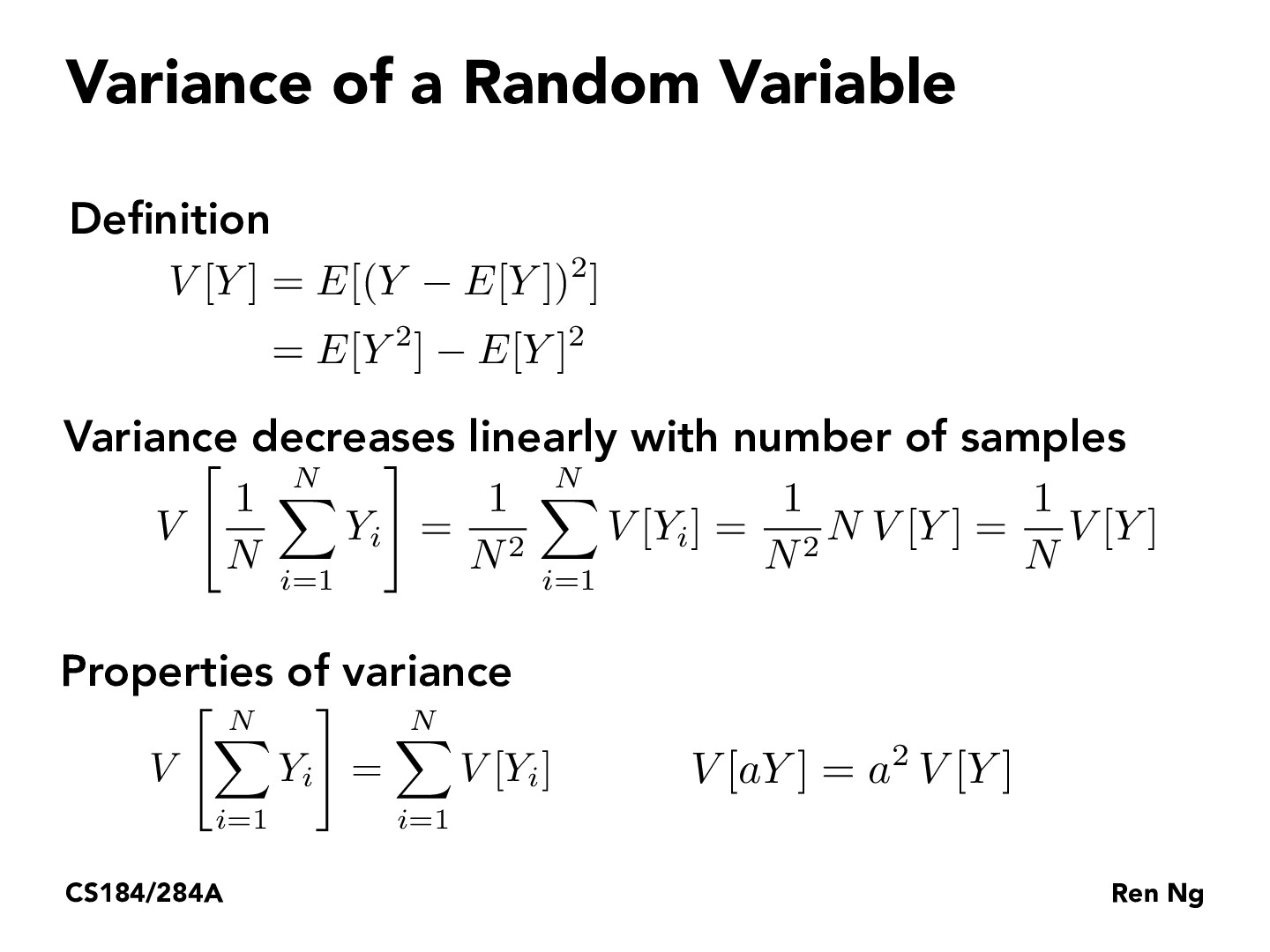Lecture 12: Monte Carlo Integration (27)JiaweiChenKodomo

Here we are assuming identical independent distributions for Yi's.JiaweiChenKodomo

[deleted]marianavazquezr

What actually is the definition of variance? Would variance of a random variable just be the difference in what each of the variable extremes can be?ellinzhao

@marianavazquezr variance is the expected squared deviation from a random variable's mean - you can see this represented in the first line under the definition of variance. not sure if that's what you were asking about though!

You must be enrolled in the course to comment